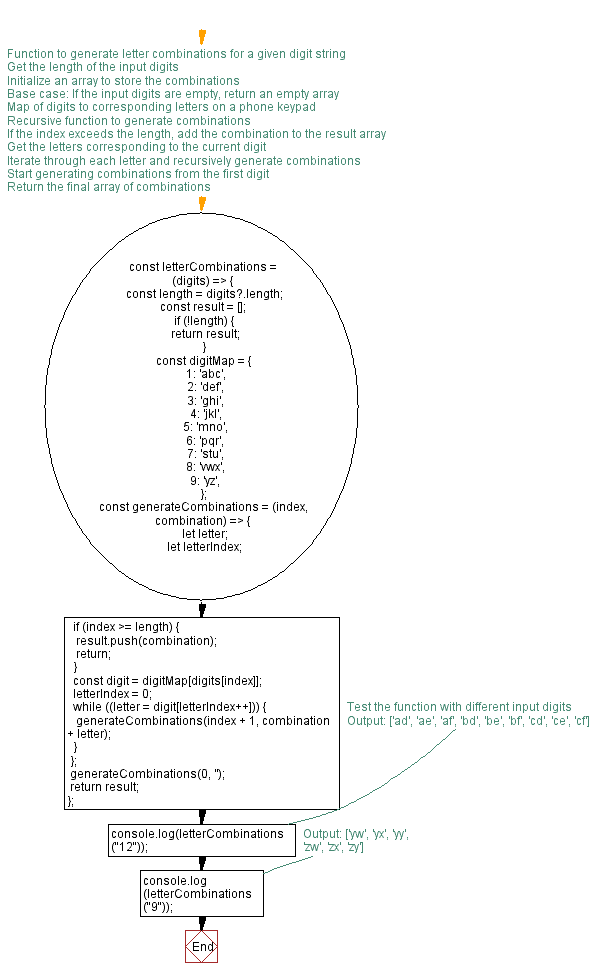# JavaScript: Letter combinations of a number

## JavaScript Function: Exercise-13 with Solution

A string consists of digits ranging from 2 to 9, inclusive. Write a JavaScript program to get all possible letter combinations that represent the number using recursion.

Letter Combination:
1: 'abc',
2: 'def',
3: 'ghi',
4: 'jkl',
5: 'mno',
6: 'pqr',
7: 'stu',
8: 'vwx'
9: 'yz'

Test Data:
("12") -> ["ad", "ae", "af", "bd", "be", "bf", "cd", "ce", "cf"]
("9") -> ["y", "z"]

Sample Solution:

HTML Code:

``````<!DOCTYPE html>
<html>
<meta charset="utf-8">
<title>Letter combinations of a number</title>
<body>

</body>
</html>
```
```

JavaScript Code:

``````const test = (digits) => {
const length = digits?.length
const result = []
if (!length) {
return result
}
const digit_Map = {
1: 'abc',
2: 'def',
3: 'ghi',
4: 'jkl',
5: 'mno',
6: 'pqr',
7: 'stu',
8: 'vwx',
9: 'yz'
}

const letter_combinations = (index, combination) => {
let letter
let letterIndex
if (index >= length) {
result.push(combination)
return
}
const digit = digit_Map[digits[index]]
letterIndex = 0
while ((letter = digit[letterIndex++])) {
letter_combinations(index + 1, combination + letter)
}
}
letter_combinations(0, '')
return result
}
console.log(test("12"))
console.log(test("9"))
```
```

Output:

```["ad","ae","af","bd","be","bf","cd","ce","cf"]
["y","z"]
```

Flowchart:Live Demo:

See the Pen javascript-recursion-function-exercise-13 by w3resource (@w3resource) on CodePen.

Improve this sample solution and post your code through Disqus

What is the difficulty level of this exercise?

Test your Programming skills with w3resource's quiz.

﻿